Courses

# Very Short Answer Type Questions- Statistics Class 10 Notes | EduRev

## Class 10 : Very Short Answer Type Questions- Statistics Class 10 Notes | EduRev

The document Very Short Answer Type Questions- Statistics Class 10 Notes | EduRev is a part of the Class 10 Course Class 10 Mathematics by VP Classes.
All you need of Class 10 at this link: Class 10

Q1. In the following distribution:

 Monthly expenditure Number of families Expenditure more than Rs 10,000 100 Expenditure more than Rs 13,000 85 Expenditure more than Rs 16,000 69 Expenditure more than Rs 19,000 50 Expenditure more than Rs 22,000 33 Expenditure more than Rs 25,000 15

The number of families having expenditure range in (Rs) 16000–19000 is:

(i) 15
(ii) 16
(iii)17
(iv) 19

Sol. (iv)1 9 [∵ 69 − 50 = 19]

Q2.  Construction of a cumulative frequency table is useful in determining the (i) mean (ii) mode (iii) median (iv) all the above

(i) mean
(ii) mode
(iii) median
(iv) all the above

Sol. (iii) median

Q3. The abscissa of the point of interaction of the ‘less than type’ and of the ‘more than type’ comutative frequency curve of grouped data gives its:

(i) mode
(ii) mean
(iii) median
(iv) All the three above

Sol. (iii) median.

Q4. For the following distribution:

 Marks Number of Students Below 10 3 Below 20 12 Below 30 27 Below 40 57 Below 50 75 Below 60 80

The modal class is

(i) 15−60
(ii) 30−40
(iii) 20−30
(iv) 10−20

Sol. (ii) 30−40

Q5. The formula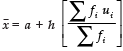is used to determine:

(i) mean
(ii) mode
(iii) median
(iv) all the three above of grouped data.

Sol. (i) mean

Q6. Consider the following distribution:

 Marks obtained Number of students More than or equal to 0 63 More than or equal to 10 58 More than or equal to 20 55 More than or equal to 30 51 More than or equal to 40 48 More than or equal to 50 42

The frequency of the class 30−40 is:

(i) 51
(ii) 48
(iii) 4
(iv) 3

Sol. (iv) 3

Q7. Fill in the blank:

Mode = (..................) − 2 (Mean)

Sol. Mode = 3 (median) − 2 (Mean)

Q8. What is the modal class of the following frequency distribution?

 Classes 0-10 10-20 20-30 30-40 40-50 50-60 Frequencies 5 8 13 38 30 4

Sol. The Highest frequency 38 corresponds to 30−40.
∴ The modal class is 30–40.

Q9. Write the empirical relation between mean, mode and median.

Sol. The three measures i.e., mean, mode and median are connected by the following empirical relation:

Mode = 3 Median – 2 Mean

Q10. Write the median class of the following distribution:

 Class Frequency 0-10 4 10-20 4 20-30 8 30-40 10 40-50 12 50-60 8 60-70 4

Sol. We have:

 Class Frequency CumulativeFrequency 0-10 4 4 + 0 = 4 10-20 4 4 + 4 = 8 20-30 8 8 + 8 = 16 30-40 10 16 + 10 = 26 40-50 12 26 + 12 = 38 50-60 8 38 + 8 = 44 60-70 4 46 + 4 = 50

Here,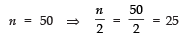∵ 25 is cumulative frequency corresponding to the class 30−40.
∴ Median class is 30−40.

Q11. What is the modal class of the following frequency distribution?

 Age (in years) 0 -10 10 - 20 20 - 30 30 - 40 40 - 50 50 - 60 Number of Patients 16 13 6 11 27 18

Sol. Here, the maximum class frequency is 27 and the class corresponding to this frequency is 40−50.
∴ The modal class is 40−50.

Q12. Find the median class of the following data:

 Marks 0 -10 10 - 20 20 - 30 30 - 40 40 - 50 50 - 60 Frequency 8 10 12 22 30 18

Sol. We have:

 Marks obtained Frequency Cumulative Frequency 0-10 8 8 + 0 = 8 10-20 10 8 + 10 = 18 20-30 12 18 + 12 = 30 30-40 22 30 + 22 = 52 40-50 30 52 + 30 = 82 50-60 18 82 + 18 = 100

Here,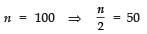∴ The median class is 30−40

Q13. Find the class marks of classes 10− 25 and 35− 55.

Sol.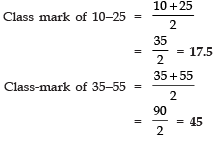Q14. What measure of central tendency is obtained graphically as the x-coordinate of the point of intersection of the two ogives for grouped data?

Sol. When two ogives intersect each other at a point and from this point, if we draw a perpendicular on the x-axis, then the point at which it cuts the x-axis gives us the median.

Q15. What is the median class of the following grouped data?

 Class Frequency 128-135 8 135-142 5 142-149 9 149-156 12 156-163 5 163-170 1

Sol. We have:

 Class Frequency Cumulative Frequency 128-135 8 8 + 0 = 8 135-142 5 5 + 8 = 13 142-149 9 9 + 13 = 22 149-156 12 12 + 22 = 34 156-163 5 5 + 34 = 39 163-170 1 1 + 39 = 40

n = 40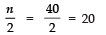⇒ The median class is 142-149.

Offer running on EduRev: Apply code STAYHOME200 to get INR 200 off on our premium plan EduRev Infinity!

132 docs

,

,

,

,

,

,

,

,

,

,

,

,

,

,

,

,

,

,

,

,

,

;